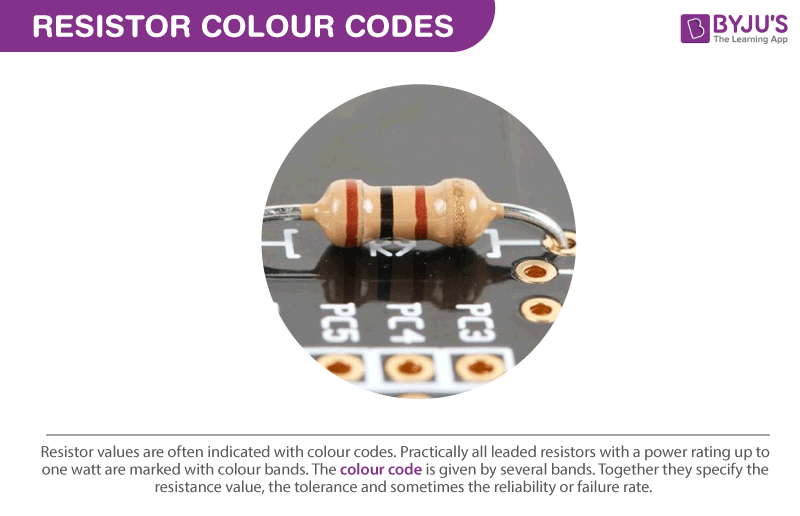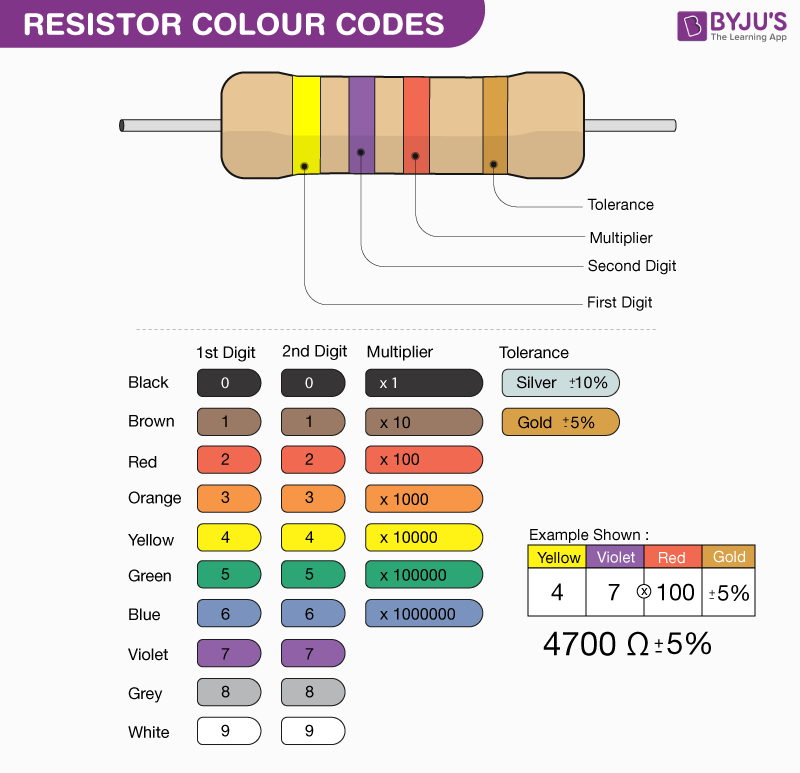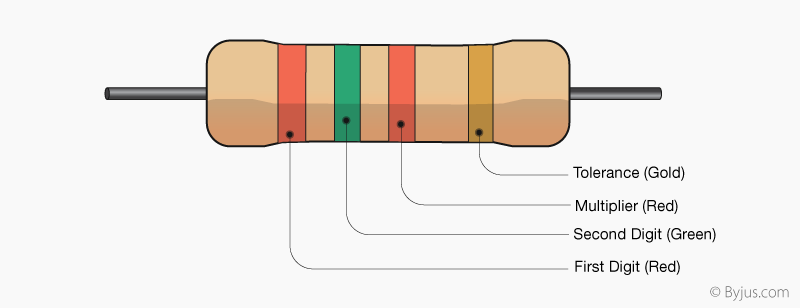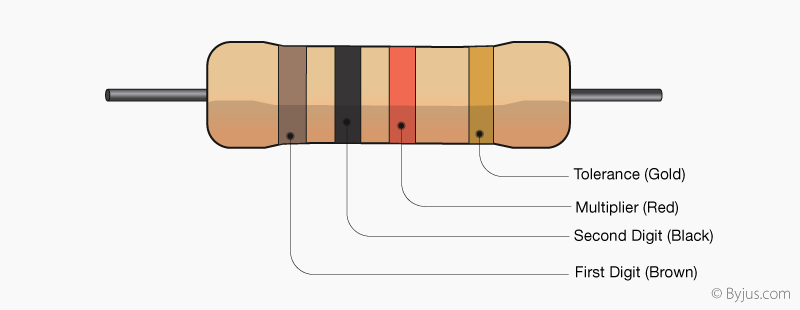# Resistor Colour Code

We know that a resistor is a two-terminal device and is an important component in building many electronic devices. The resistor is a component that is used to limit or regulate the flow of electric current. We commonly see resistors printed with different colours. It usually contains four bands of colours. To know the value of resistors, one must know how to calculate the resistance colour code. In this article, let us learn what is resistance colour code, how to read resistor colour code, and examples to find resistance colour code.

## What is Resistor Colour Code?

Resistors are usually very tiny, and it is challenging to print resistance values on them. So, colour bands are printed on them to represent the electrical resistance. These colour bands are known as resistor colour codes. The resistor colour code was invented in the 1920s by the Radio Manufacturers Association (RMA).All leaded resistors with a power rating up to one watt are marked with colour bands. They are given by several bands and together they specify the resistance value, the tolerance rate and sometimes the reliability or failure rates. The number of bands present in a resistor varies from three to six. The first two bands indicate the resistance value and the third band serves as a multiplier. In this piece of article, let us discuss how to read resistor colour codes, look at an example and learn a mnemonic to remember the number sequence.

Uses of Resistor

 For Better Understanding: What is a Resistor? Types of Resistor

## Resistor Colour Table

 Colour Digit Multiplier Tolerance Black 0 1 Brown 1 10 ± 1% Red 2 100 ± 2% Orange 3 1000 Yellow 4 10,000 Green 5 100,000 ± 0.5% Blue 6 1,00,000 ± 0.25% Violet 7 10,000,000 ± 0.1% Grey 8 ± 0.05% White 9 Gold 0.1 ± 5% Silver 0.01 ± 10%

## How to Read Resistor Colour Code?

• To read them, hold the resistor such that the tolerance band is on your right. The tolerance band is usually gold or silver in colour and is placed a little further away from the other bands.
• Starting from your left, note down all the colours of the bands and write them down in sequence.
• Next, use the table given below to see which digits they represent.
• The band just next to the tolerance band is the multiplier band. So if the colour of this band is Red (representing 2), the value given is 102.### Learning with an example

After learning about resistance colour codes, let us learn how to find resistor colour codes with an example. Here’s an example to get you started:The band colours for resistor colour code in the order:

 Band colours in order RED RED BLACK GOLD Digit representation 2 2 1 ±5% Value 22 Ω ±5 %

The tolerance values represent by how much the resistance can vary from its mean value in terms of percentage. A gold band represents the lowest variation, so be sure to buy these at the electronics store.  The value of the given resistance is: 22 Ω ± 5%. The tolerance of the resistor can be calculated as follows:

Tolerance=Value of resistor × value of tolerance band

= 22 Ω × 5% = 1.1 Ω

This means that the 22 Ω resistor with a tolerance value of 1.1 Ω could range from the actual value as much as 23.1 Ω to as little as 20.9 Ω. It is important to note that the band next to the tolerance band represents the multiplier. All the bands to the left of this band represent the significant digits. There can be more than two such bands.

## The below video helps in understanding why colour coding is important in resistors### Resistor Colour Code Mnemonic

You can remember the colour code values using the following mnemonic:

BB ROY of Great Britain had a Very Good Wife

The capital letters represent the first letters of the colours and their positions the digit values. Now that we have an idea to determine the resistance value of a given resistor. Let us solve some problems related to this in the next section.

## Resistor Colour Code Examples

Q1) Determine the resistance of the given resistor with the given colour sequence (Red, Green, Red, Gold).

As we know, the first two colours represent the significant digits of resistance value so the given colours represent digits 2 and 5. The third band is a multiplier band. Hence, the colour red represents a multiplier factor of 102. The last band represents the tolerance level and the tolerance level of the resistor is ±5%. Hence, the resistance value of the given resistor is 2500 ± 5% Ω or 2.5 kΩ.Q2) What colour bands would a resistor of resistance value 1000 Ω with a tolerance level ±5% have?
The colour bands of the resistor would look like this:## Learn the factors that affect electrical resistance by watching the video below## Frequently Asked Questions – FAQs

Q1

### How does a resistor colour code work?

The resistor bands are made of several colour bands which together specify the resistance value, tolerance and sometimes the reliability rate. Resistors with high precision have five bands. The first three bands indicate the significant digits, the fourth band is the multiplying factor and the fifth band represents the tolerance.
Q2

### Why are resistors colour coded?

Resistors are normally tiny, and it is difficult to print resistor values on them. So, colour bands are printed on them to represent the electrical resistance.
Q3

### How to remember the resistor colour code?

The resistor colour codes can be remembered with the help of mnemonic device “BB ROY of Great Britain had a Very Good Wife,” the capital letters represent the first letters of the colours and their positions the digit values.
Q4

### What is the resistor colour code for a 1000 Ω resistor?

A 1000 Ω resistor has a four-band colour code following a colour sequence of Brown, Black, Red and Gold. The 1000 Ω resistor has a mulitplying factor of 100 (Red) and a tolerance level of ±5% (Gold).
Q5

### Who invented the resistor colour code?

The colour code was developed in the 1920s by the Radio Manufacturers Association (RMA).

## Watch the full revision of the chapter Magnetic Effects of Electric Current Class 10Hope you learned how to find the resistance colour code. Stay tuned to BYJU’S and Fall in love with learning!

Test your Knowledge on Resistor Colour Codes